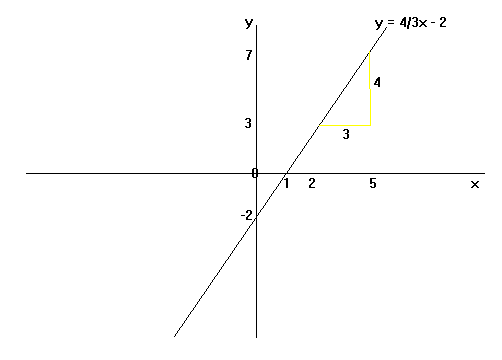## Equation of a Straight Line

Equations of straight lines are in the form y = mx + c (m and c are numbers). m is the gradient of the line and c is the y-intercept (where the graph crosses the y-axis).

NB1: If you are given the equation of a straight-line and there is a number before the 'y', divide everything by this number to get y by itself, so that you can see what m and c are.
NB2: Parallel lines have equal gradients.The above graph has equation y = (4/3)x - 2 (which is the same as 3y + 6 = 4x).
Gradient = change in y / change in x = 4 / 3
It cuts the y-axis at -2, and this is the constant in the equation.

This video shows you how to calculate the equation of a straight line given two points